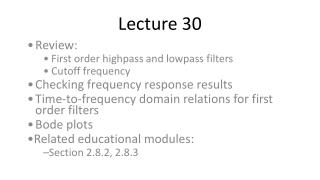Download PresentationLecture 30

Lecture 30 - PowerPoint PPT Presentation

Lecture 30. Review: First order highpass and lowpass filters Cutoff frequency Checking frequency response results Time-to-frequency domain relations for first order filters Bode plots Related educational modules: Section 2.8.2, 2.8.3. Filters.I am the owner, or an agent authorized to act on behalf of the owner, of the copyrighted work described.
Download PresentationLecture 30

Download Policy: Content on the Website is provided to you AS IS for your information and personal use and may not be sold / licensed / shared on other websites without getting consent from its author.While downloading, if for some reason you are not able to download a presentation, the publisher may have deleted the file from their server.

- - - - - - - - - - - - - - - - - - - - - - - - - - E N D - - - - - - - - - - - - - - - - - - - - - - - - - -
Presentation Transcript
1. Lecture 30 Review: First order highpass and lowpass filters Cutoff frequency Checking frequency response results Time-to-frequency domain relations for first order filters Bode plots Related educational modules: Section 2.8.2, 2.8.3

2. Filters • Circuits categorized by their amplitude response • Filters “pass” some frequencies and “stop” others • Lowpass filters pass low frequencies • Highpass filters pass high frequencies • Filters are also categorized by their order • The filter order corresponds to the order of the circuit’s governing differential equation • Circuits I: only first order low- and highpass filters

3. First order filters • Review: Low- and highpass filter response examples • Cutoff frequency separates the pass- and stopbands • Where the amplitude response is times the max amplitude

4. Annotate previous slide to show cutoff frequencies • Note that cutoff frequency is related to maximum gain of filter • Change max gain on figure (write in) => gain at corner frequency changes

5. Time vs. frequency domain characterization • In the time domain, we characterized first order circuits by their step response • DC gain, time constant ( ) • We can likewise characterize first order circuits by their frequency response • DC gain, cutoff frequency (c)

6. On previous slide, sketch step response, show DC gain • Then show that cosine input becomes a step input as frequency -> zero

7. Time-to-Frequency domain relations – cont’d • Governing differential equation for first order system: • Converting to frequency domain:

8. On previous slide, show: • (1) time domain DC gain, tau • (2) conversion to frequency response => u(t)=Ue^(jwt), etc. • (3) freqency response -> magnitude response • (4) DC gain and cutoff frequency from mag. Resp.

9. Checking amplitude responses • We can (fairly) easily check our amplitude responses at very low and very high frequencies • Capacitors, inductors replaced by open, short circuits • Results in purely resistive network • Analyze resistive network, and compare result to amplitude response • Provides “physical insight” into low, high frequency operation

10. Inductors at low, high frequencies • Inductor impedance: •  = 0  • Inductor behaves like short circuit at low frequencies •     • Inductor behaves like open circuit at high frequencies

11. Capacitors at low, high frequencies • Capacitor impedance: •  = 0  • Capacitor behaves like open circuit at low frequencies •     • Capacitor behaves like short circuit at high frequencies

12. Example – checking amplitude response • What is the amplitude response of the circuit below as 0 and  ?

13. Bode plots – introduction • We have used linear scales to plot frequency responses • Selective use of logarithmic scales has a number of advantages: • Amplitudes and frequencies tend to span large ranges • Logarithms convert multiplication and division to addition and subtraction • Human senses work logarithmically • Bode plots use logarithmic scales to simplify plotting of frequency responses and interpretation of plots

14. Do demos on previous slide • Note large ranges of frequencies; sensitivity to frequency ranges • Note human senses work logarithmically

15. Nomenclature relative to log scales • Logarithmic scales convert multiplicative factors to linear differences • Some of these multiplicative factors have special names • A factor of 10 change in frequency is a decade difference on a log scale • A factor of 2 change in frequency is an octave difference on a log scale

16. Bode Plots • Bode plots are a specific format for plotting frequency responses • Frequencies are on logarithmic scales • Amplitudes are on a decibel (dB) scale • Phases are on a linear scale

17. Decibels • Named for Alexander Graham Bell • Common gains and their decibel values: 10  20dB 1  0dB 0.1  -20dB 0.5  -6dB  -3dB

18. Bode plots of first order filters • Frequency response for typical first order lowpass filter: • Magnitude, phase responses:

19. Bode plot – magnitude response

20. Annotate previous slide to show asymptotic behavior, -3dB point at wc, intersection of asymptotes is at wc • Note that I can muliply this by a gain, without affecting the shape of the curve – it simply moves up and down

21. Bode plot – phase response

22. Example • Sketch a Bode plot for the circuit below.